## Overview: NLP Solver

The sparse nonlinear programming (NLP) solver is a component of the OPTMODEL procedure that can solve optimization problems containing both nonlinear equality and inequality constraints. The general nonlinear optimization problem can be defined as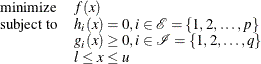where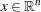is the vector of the decision variables;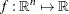is the objective function;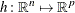is the vector of equality constraints—that is,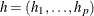;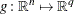is the vector of inequality constraints—that is,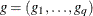; and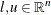are the vectors of the lower and upper bounds, respectively, on the decision variables.

It is assumed that the functions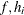, and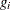are twice continuously differentiable. Any point that satisfies the constraints of the NLP problem is called a feasible point, and the set of all those points forms the feasible region of the NLP problem—that is,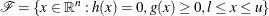.

The NLP problem can have a unique minimum or many different minima, depending on the type of functions involved. If the objective function is convex, the equality constraint functions are linear, and the inequality constraint functions are concave, then the NLP problem is called a convex program and has a unique minimum. All other types of NLP problems are called nonconvex and can contain more than one minimum, usually called local minima. The solution that achieves the lowest objective value of all local minima is called the global minimum or global solution of the NLP problem. The NLP solver can find the unique minimum of convex programs and a local minimum of a general NLP problem. In addition, the solver is equipped with specific options that enable it to locate the global minimum or a good approximation of it, for those problems that contain many local minima.

The NLP solver implements the following primal-dual methods for finding a local minimum:

• interior point trust-region line-search algorithm

• active-set trust-region line-search algorithm

Both methods can solve small-, medium-, and large-scale optimization problems efficiently and robustly. These methods use exact first and second derivatives to calculate search directions. The memory requirements of both algorithms are reduced dramatically because only nonzero elements of matrices are stored. Convergence of both algorithms is achieved by using a trust-region line-search framework that guides the iterations towards the optimal solution. If a trust-region subproblem fails to provide a suitable step of improvement, a line-search is then used to fine tune the trust-region radius and ensure sufficient decrease in objective function and constraint violations.

The interior point technique implements a primal-dual interior point algorithm in which barrier functions are used to ensure that the algorithm remains feasible with respect to the bound constraints. Interior point methods are extremely useful when the optimization problem contains many inequality constraints and you suspect that most of these constraints will be satisfied as strict inequalities at the optimal solution.

The active-set technique implements an active-set algorithm in which only the inequality constraints that are satisfied as equalities, together with the original equality constraints, are considered. Once that set of constraints is identified, active-set algorithms typically converge faster than interior point algorithms. They converge faster because the size and the complexity of the original optimization problem can be reduced if only few constraints need to be considered.

For optimization problems that contain many local optima, the NLP solver can be run in multistart mode. If the multistart mode is specified, the solver samples the feasible region and generates a number of starting points. Then the local solvers can be called from each of those starting points to converge to different local optima. The local minimum with the smallest objective value is then reported back to the user as the optimal solution.

The NLP solver implements many powerful features that are obtained from recent research in the field of nonlinear optimization algorithms (Akrotirianakis and Rustem, 2005; Armand, Gilbert, and Jan-Jégou, 2002; Erway, Gill, and Griffin, 2007; Forsgren and Gill, 1998; Vanderbei, 1999; Wächter and Biegler, 2006; Yamashita, 1998). The term primal-dual means that the algorithm iteratively generates better approximations of the decision variables x (usually called primal variables) in addition to the dual variables (also referred to as Lagrange multipliers). At every iteration, the algorithm uses a modified Newton’s method to solve a system of nonlinear equations. The modifications made to Newton’s method are implicitly controlled by the current trust-region radius. The solution of that system provides the direction and the steps along which the next approximation of the local minimum is searched. The active-set algorithm ensures that the primal iterations are always within their bounds—that is,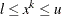, for every iteration k. However, the interior approach relaxes this condition by using slack variables, and intermediate iterations might be infeasible.